• 一、矩阵求导   一般来讲，我们约定x=(x1,x2,...xN)Tx=(x1,x2,...xN)Tx=(x_1,x_2,...x_N)^T，这是分母布局。常见的矩阵求导方式有：向量对向量求导，标量对向量求导，向量对标量求导。 1、向量对向量求导 2、...
一、矩阵求导

一般来讲，我们约定x=(x1,x2,...xN)T$x=\left({x}_{1},{x}_{2},...{x}_{N}{\right)}^{T}$$x=(x_1,x_2,...x_N)^T$，这是分母布局。常见的矩阵求导方式有：向量对向量求导，标量对向量求导，向量对标量求导。

1、向量对向量求导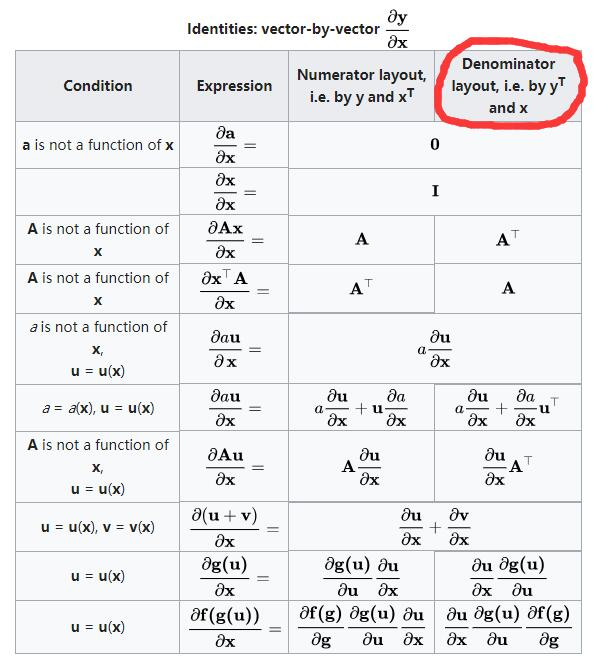2、标量对向量求导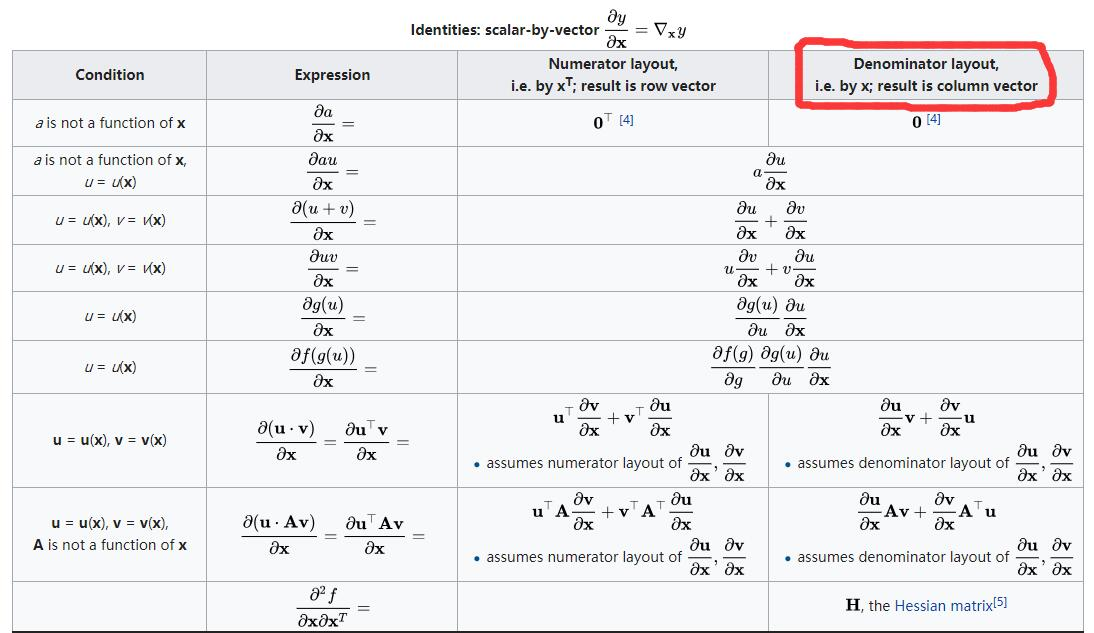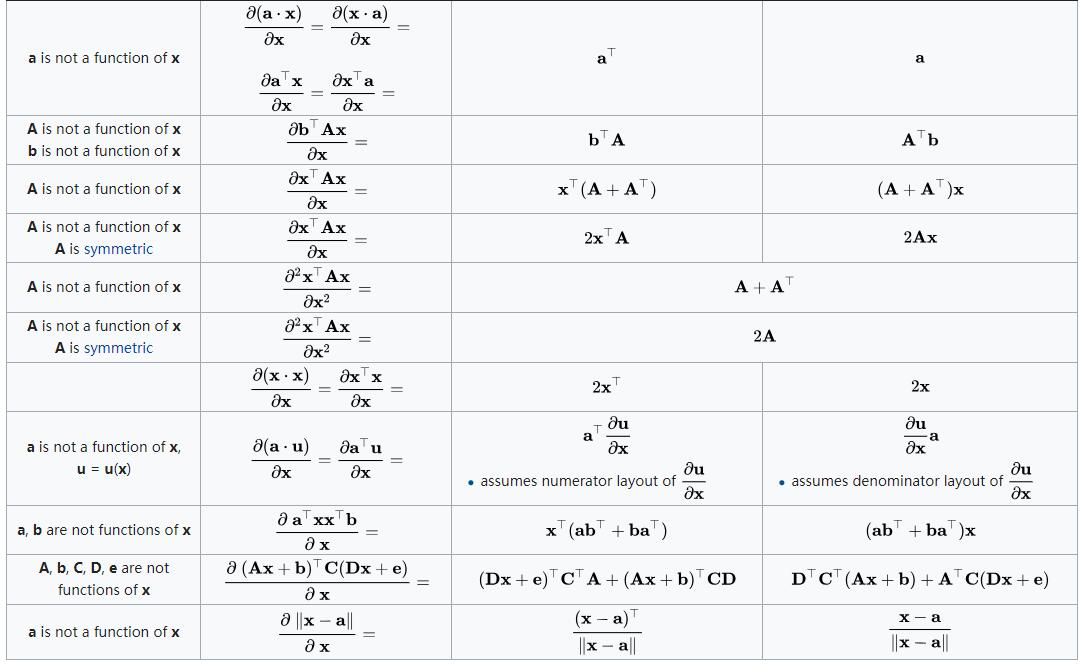3、向量对标量求导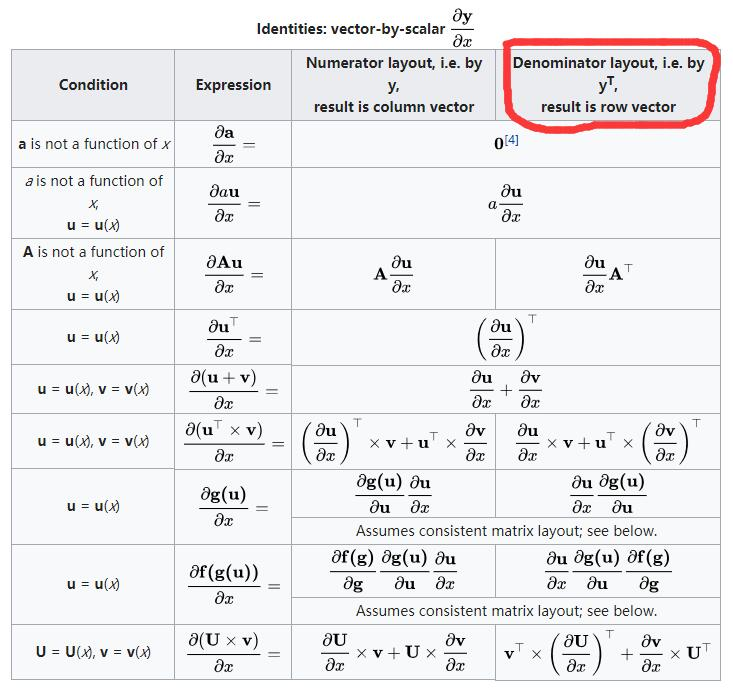其他的可以参考wiki：维基百科矩阵求导公式

二、几种重要的矩阵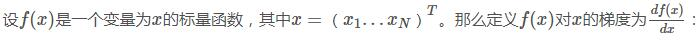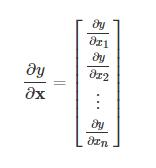2、雅克比矩阵（Jacobian matrix）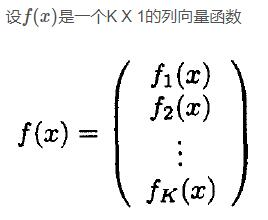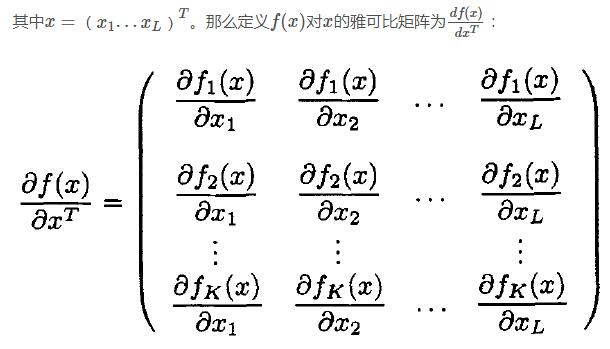3、海森矩阵（Hessian matrix）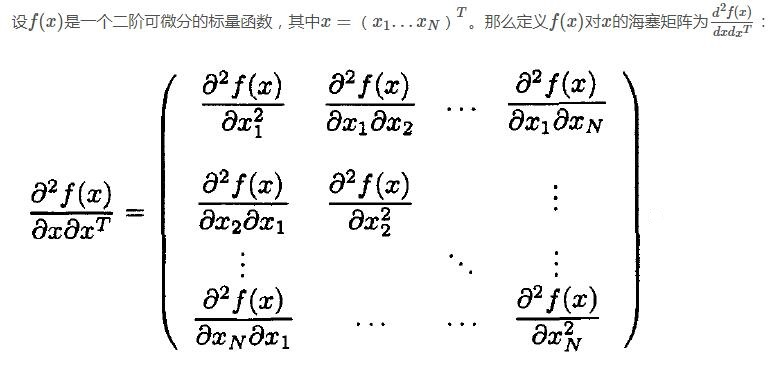三、常用的矩阵求导公式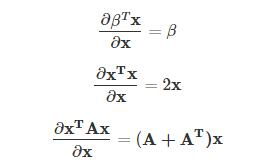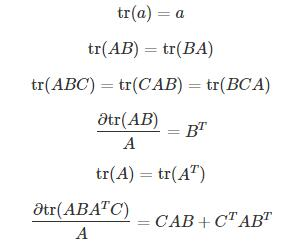参考：
https://blog.csdn.net/xtydtc/article/details/51133903
https://blog.csdn.net/yc461515457/article/details/49682473
展开全文梯度 雅克比矩阵 海森矩阵
• 机器学习门槛之矩阵求导（二）行云流水秀：机器学习门槛之矩阵求导（二）​zhuanlan.zhihu.com提到有六种矩阵求导的形式如下：矩阵求导形式总结 1： ，就是高中所学的那些求导公式2： 3： 4: 5: 6： 常用的矩阵求导...
机器学习门槛之矩阵求导（二）行云流水秀：机器学习门槛之矩阵求导（二）​zhuanlan.zhihu.com提到有六种矩阵求导的形式如下：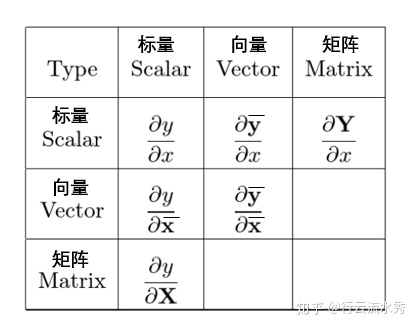矩阵求导形式总结 1：，就是高中所学的那些求导公式2：3：4:5:6：常用的矩阵求导公式(采用分子布局的矩阵求导公式)：下面的式子中小写字母a表示标量a，带横线的小写字母表示列向量，大写字母A表示矩阵，a，和A都不是x或的函数。需要记住 下面的求导公式，就像记住高中常见的求导公式一样。(c1)(是与相同规模的列向量)(c2)(是与相同规模的行向量)(c3)(是与相同规模的矩阵)(c4)(是矩阵)(c5)(是单位矩阵)下面的几个最重要：下面的式子中小写字母a表示标量a，带横线的小写字母表示列向量，大写字母A表示矩阵，a，和A都不是x或的函数。(c6)(c7)(c8)(c9)(c10)(c11)回到多元线性回归模型：行云流水秀：机器学习门槛之矩阵求导（一）​zhuanlan.zhihu.com行云流水秀：机器学习门槛之矩阵求导（二）​zhuanlan.zhihu.com下面的式子中小写字母a表示标量a，带横线的小写字母表示列向量，大写字母A表示矩阵，a，和A都不是x或的函数。所以loss对求导转化为了以上四个式子对求导：(1)，因为和无关(2)根据公式（c6）可知就是公式c6的，故(3)根据公式（c6）可知：就是公式里的，(4)根据公式（c11):可知就是公式c11里的A：综上(1)(2)(3)(4)可知：大功告成！多元线性回归或者最小二乘法（最小平方法）的解为：等号两边同时转置得：所以可得：，这就是多元线性回归的解。大功彻底告成！总结：矩阵求导要会套公式，和高中数学求导一样。去哪里找矩阵求导公式，一个是 The Matrix Cookbook ，一个小册子。下载链接：链接：https://pan.baidu.com/s/12_Xqlw1VWosEOpwqB_S1SA 提取码：71md二是Matrix Calculus网站Matrix Calculus​www.matrixcalculus.org，使用参考：二圈妹：矩阵求导简介​zhuanlan.zhihu.com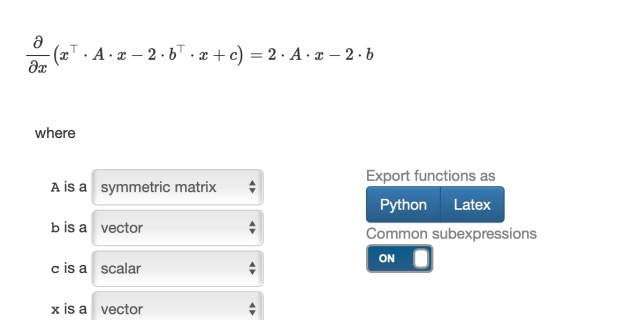展开全文• 记录下几个比较好的矩阵求导 机器学习中的线性代数之矩阵求导 https://blog.csdn.net/u010976453/article/details/54381248 通过一个例子快速上手矩阵求导 ... 矩阵求导简要笔记 ...
记录下几个比较好的矩阵求导
机器学习中的线性代数之矩阵求导
https://blog.csdn.net/u010976453/article/details/54381248
通过一个例子快速上手矩阵求导
矩阵求导简要笔记
https://blog.csdn.net/u012151283/article/details/71056029
维基百科里有相应的表格可以查找：需要翻墙
https://en.wikipedia.org/wiki/Matrix_calculus#Scalar-by-vector_identities


展开全文矩阵 线性代数 机器学习
• ## 机器学习中的线性代数之矩阵求导

万次阅读 多人点赞 2017-01-12 19:57:57
接下来针对机器学习公式推导过程中经常用到的矩阵求导，我们做一个详细介绍。矩阵求导（Matrix Derivative）也称作矩阵微分（Matrix Differential），在机器学习、图像处理、最优化等领域的公式推导中经常用到。
前面针对机器学习中基础的线性代数知识，我们做了一个常用知识的梳理。接下来针对机器学习公式推导过程中经常用到的矩阵求导，我们做一个详细介绍。
矩阵求导（Matrix Derivative）也称作矩阵微分（Matrix Differential），在机器学习、图像处理、最优化等领域的公式推导中经常用到。
矩阵的微积分本质上是多元变量的微积分问题，只是应用在矩阵空间上而已
根据$\bf Y$ 与 $\bf X$ 的不同类型（实值、向量、矩阵）给出如下表中的表示：

类型
标量（Scalar）$y$
向量（Vector）$\bf y$
矩阵（Matrix）$\bf Y$

Scalar $x$
$\frac {\partial y}{\partial x}$
$\frac {\partial \bf y}{\partial x}$
$\frac {\partial \bf Y}{\partial x}$

Vector $\bf x$
$\frac {\partial y}{\partial \bf x}$
$\frac {\partial \bf y}{\partial \bf x}$

Matrix $\bf X$
$\frac {\partial y}{\partial \bf X}$

下面我们根据分子的布局（即X的类型）来介绍矩阵的导数求解
0 布局约定（Layout conventions）
事实上，所有求导的法则都可以从最基本的求导规则推导出来。不知你有没发现，不同的文献中，同样的式子求导的结果有时候会不一样，仔细观察会发现刚好相差一个转置，于是我们得先说说求导的两个派别（布局）。
由向量关于向量的求导$\frac{\partial y}{\partial x}$可以得出两种矛盾的表示：结果表示为$n×m$ 矩阵或$m×n$ 矩阵。也就是把$\bf y$ 表示为列向量$\bf x$ 表示为行向量或者反过来表示的问题。
布局（Layout）：在矩阵求导中有两种布局，分别为分母布局(denominator layout)和分子布局(numerator layout)。这两种不同布局的求导规则是不一样的。
向量 ${\bf y} = \begin {bmatrix} y_1 \\ y_2\\ \vdots \\ y_n\end{bmatrix}$，关于标量$x$ 的求导，
在分子布局下，为：
$\frac {\partial \bf y}{\partial x} = \begin {bmatrix} \frac{\partial y_1}{\partial x} \\ \frac{\partial y_2}{\partial x} \\ \vdots \\ \frac{\partial y_n}{\partial x} \end{bmatrix}\tag{1}$
而在分母布局下，为：
$\frac {\partial \bf y}{\partial x} = \begin {bmatrix} \frac{\partial y_1}{\partial x} & \frac{\partial y_2}{\partial x} & \cdots & \frac{\partial y_n}{\partial x} \end{bmatrix}\tag{2}$
通过观察和推导我们可以知道，分子布局和分母布局之间刚好差一个转置，即在分子布局下与原来$\bf Y$相同，而在分母布局下差一个转置。
对于正切矩阵$\frac{\partial y}{\partial x}$采用分母布局，即$\bf Y^ \top$，很不符合表达的习惯，所以本文中我们采用的是分子布局。
1 关于标量的导数
对于 $\bf X$  是标量的情况，是我们最熟悉的一种情况。
1.1 标量关于标量X的求导
这中情况就是我们平时的代数求导，直接就是$\frac {\partial y}{\partial x}$
1.2 向量关于标量X的求导
向量 ${\bf y} = \begin {bmatrix} y_1 \\ y_2\\ \vdots \\ y_n\end{bmatrix}$，关于标量$x$ 的求导就是 ${\bf y}$ 的每一个元素分别对$x$求导，可以表示为
$\frac {\partial \bf y}{\partial x} = \begin {bmatrix} \frac{\partial y_1}{\partial x} \\ \frac{\partial y_2}{\partial x} \\ \vdots \\ \frac{\partial y_n}{\partial x} \end{bmatrix}\tag{3}$
此时为正切向量，$\frac {\partial \bf y}{\partial x}$ 为 $\bf y$ 的正切向量，有映射 $\bf y$ : ${\Bbb R}^m \implies {\Bbb R}^m$ 。
1.3 矩阵关于标量X的求导
矩阵对标量的求导类似于向量关于标量的求导，也就是矩阵的每个元素分别对标量$x$求导，矩阵 ${\bf Y} = \begin {bmatrix} y_{11} & y_{12} & \cdots & y_{1n} \\ y_{21} & y_{22} & \cdots & y_{2n} \\ \vdots & \vdots & \ddots & \vdots \\ y_{n1} & y_{n2} & \cdots & y_{nn} \end{bmatrix}$ 对标量$x$的导数为
$\frac {\partial \bf Y}{\partial x} = \begin {bmatrix} \frac{\partial y_{11} }{\partial x } & \frac{\partial y_{12} }{\partial x }& \cdots & \frac{\partial y_{1n} }{\partial x } \\ \frac{\partial y_{21}}{\partial x } & \frac{\partial y_{22}}{\partial x } & \cdots & \frac{\partial y_{2n}}{\partial x } \\ \vdots & \vdots & \ddots & \vdots \\ \frac{\partial y_{n1} }{\partial x } & \frac{\partial y_{n2} }{\partial x } & \cdots & \frac{\partial y_{nn}}{\partial x } \end{bmatrix}\tag{4}$
2 关于向量的导数
2.1标量关于向量 $\bf x$ 的导数
标量$y$ 关于向量 ${\bf x } = \begin {bmatrix} x_1 \\ x_2\\ \vdots \\ x_n\end{bmatrix}$ 的求导可以表示为
$\frac {\partial y}{\partial \bf x} = \begin {bmatrix} \frac{\partial y}{\partial x_{1} }\ \frac{\partial y}{\partial x_{2} } \ \cdots \ \frac{\partial y}{\partial x_{n} } \end{bmatrix}\tag{5}$
此时的向量叫做梯度向量。 $\frac {\partial y}{\partial \bf x}$ 为标量$y$ 在空间 ${\Bbb R}^n$ 的梯度，该空间以$x$ 为基。
2.2 向量关于向量 $\bf x$ 的导数
向量函数（即函数组成的向量）${\bf y} = \begin {bmatrix} y_1 \\ y_2\\ \vdots \\ y_n\end{bmatrix}$ 关于向量${\bf x } = \begin {bmatrix} x_1 \\ x_2\\ \vdots \\ x_n\end{bmatrix}$  的导数记作
$\frac {\partial \bf y}{\partial \bf x} = \begin {bmatrix} \frac{\partial y_{1} }{\partial x_{1} } & \frac{\partial y_{1} }{\partial x_{2} }& \cdots & \frac{\partial y_{1} }{\partial x_{n} } \\ \frac{\partial y_{2}}{\partial x_{1} } & \frac{\partial y_{2}}{\partial x_{2} } & \cdots & \frac{\partial y_{2}}{\partial x_{n} } \\ \vdots & \vdots & \ddots & \vdots \\ \frac{\partial y_{n} }{\partial x_{1} } & \frac{\partial y_{n} }{\partial x_{2} } & \cdots & \frac{\partial y_{n}}{\partial x_{n} } \end{bmatrix}\tag{6}$
此时获得的矩阵$\frac {\partial \bf y}{\partial \bf x}​$ 叫做Jacobian 矩阵。
2.3 矩阵关于向量 $\bf x$ 的导数
矩阵 ${\bf Y} = \begin {bmatrix} y_{11} & y_{12} & \cdots & y_{1n} \\ y_{21} & y_{22} & \cdots & y_{2n} \\ \vdots & \vdots & \ddots & \vdots \\ y_{n1} & y_{n2} & \cdots & y_{nn} \end{bmatrix}$ 对向量${\bf x } = \begin {bmatrix} x_1 \\ x_2\\ \vdots \\ x_n\end{bmatrix}$ 的导数是推导中最复杂的一种，我们可以表示为
$\frac {\partial \bf Y}{\partial \bf x} = \begin {bmatrix} \frac{\partial y_{11} }{\partial x_{1} } & \frac{\partial y_{1n} }{\partial x_{2} }& \cdots & \frac{\partial y_{1n} }{\partial x_{n} } \\ \frac{\partial y_{21}}{\partial x_{1} } & \frac{\partial y_{22}}{\partial x_{2} } & \cdots & \frac{\partial y_{2n}}{\partial x_{n} } \\ \vdots & \vdots & \ddots & \vdots \\ \frac{\partial y_{n1} }{\partial x_{1} } & \frac{\partial y_{n2} }{\partial x_{2} } & \cdots & \frac{\partial y_{nn}}{\partial x_{n} } \end{bmatrix}\tag{7}$
3 关于矩阵的导数
我们一般只考虑标量关于矩阵的导数（因为矩阵对向量和矩阵的导数与前面2.3节的内容一致或相似），即标量$y$ 对矩阵 $\bf X$ 的导数为 $\frac {\partial y}{\partial \bf X}$ ，此时的导数是梯度矩阵，可以表示为下式：
$\frac {\partial y}{\partial \bf X} =\begin {bmatrix} \frac{\partial y }{\partial x_{11} } & \frac{\partial y }{\partial x_{21} }& \cdots & \frac{\partial y }{\partial x_{n1} } \\ \frac{\partial y}{\partial x_{12} } & \frac{\partial y}{\partial x_{22} } & \cdots & \frac{\partial y}{\partial x_{n2} } \\ \vdots & \vdots & \ddots & \vdots \\ \frac{\partial y }{\partial x_{1n} } & \frac{\partial y }{\partial x_{2n} } & \cdots & \frac{\partial y}{\partial x_{nn} } \end{bmatrix}\tag{8}$
4 维度分析
当我们对一些复杂的矩阵乘积求偏导的时候，直接求很难直接求出，这时候我们可以通过分析矩阵的维度来得到结果。例如:
考虑以下导数 $\frac {\partial \bf Au}{\partial \bf x}$ ，其中 $\bf A$ 与$\bf x$ 无关 且有 ${\bf A} \in {\Bbb R}^ {m \times n}$， ${\bf u } \in {Bbb R}^{n \times 1}$ ，${\bf x} \in {\Bbb R}^{p \times 1}$，我们知道结果肯定和$\frac {\partial \bf u}{\partial \bf x}$  有关，于是先把  $\bf A$ 提出求导式，至于到了哪暂时不知道，接着我们知道 $\frac {\partial \bf u}{\partial \bf x} \in {\Bbb R}^ {p \times n}$，于是 $\bf A$ 只能转置后添加到后面。因此有
$\frac {\partial \bf Au}{\partial \bf x} = \frac {\partial \bf u}{\partial \bf x} {\bf A}^ \top \tag{9}$
再考虑问题 $\frac {\partial \bf x^\top A x}{\partial \bf x}$ ，其中 ${\bf A} \in {\Bbb R}^ {n \times n}$， ${\bf x } \in {\Bbb R}^{n \times n}$ ,
为了分析这个问题我们考虑一个更一半的问题
$\frac {\partial \bf x^\top A x}{\partial \bf x}\tag{10}$
其中 ${\bf A} \in {\Bbb R}^ {n \times n}$， ${\bf x } \in {Bbb R}^{n \times n}$，且 $\bf A$ 与$\bf x$ 和 $\bf y$ 无关 。于是我们利用维度分析，采用非精确的乘积法则，可以将它分为两个部分
$\frac {\partial \bf (x^\top A) y}{\partial \bf x} \tag{11}$
于是结果与两部分相关，一个是
$\frac {\partial \bf y}{\partial \bf x} \in {\Bbb R}^ {m \times n} \tag{12}$
另一个是
$\frac {\partial \bf x^\top A}{\partial \bf x} = {\bf A} \in {\Bbb R}^ {m \times n} \tag{13}$
同样通过维度分析，我们可以得到
$\frac {\partial \bf (x^\top A) y}{\partial \bf x} = \frac {\partial \bf y}{\partial \bf x} \bf A^\top \bf x + \bf A \bf y \tag{14}$
因此经过维度的比较我们可以得到
$\frac {\partial \bf x^\top A x}{\partial \bf x} = (\bf A^\top + A)x\tag{14}$
通过以上两个示例的学习，我们可以知道在求解复杂矩阵的求导问题时，通过维度来判断矩阵的导数形式很简便同时也不容易出错。下图是机器学习中常见的矩阵求导形式，可供参考：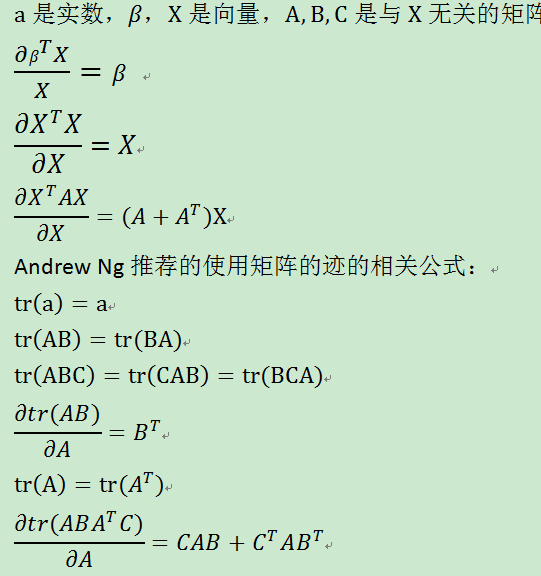5 总结
在本文中，我们针对机器学习推导中的矩阵求导问题做了一个全面的分析，同时结合前文 深度学习系列（二）——机器学习中的线性代数知识 介绍的机器学习中线性代数的基础知识，我们对线性代数部分做了详细的了解。下一章我们介绍机器学习中涉及到的概率知识。


展开全文机器学习 线性代数 矩阵 matrix...